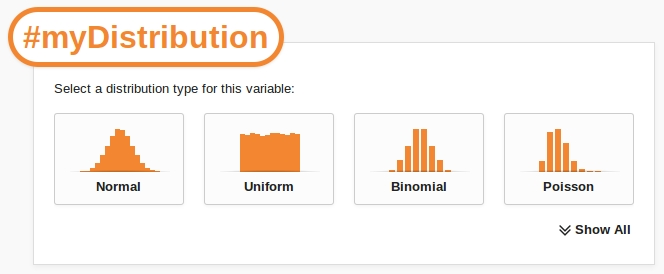### Input

Caladis makes it easy to perform calculations involving probability distributions. To perform a calculation, enter a mathematical expression in the input box on the home page. Mathematical expressions can include numbers, operators, functions and constants (see INPUT EXPRESSIONS for more details). A simple expression might take the form:

1 + 2

This expression does not include any probability distributions so will have a single solution (in this case 3). To make our expression probabilistic we must add a probability distribution variable. These are defined using the hash symbol, #, and can include upper-case letters, lower-case letters and numbers 0-9. Adding to our previous example, we define a distribution variable called "myDistribution":

1 + 2 + #myDistribution

As we type the variable's name, Caladis produces a popup enabling us to define what type of probability distribution our variable is:The popup lists all of the available probability distributions in Caladis. To select a distribution type simply click on its icon.When a distribution is selected (in this case the Normal distribution), the content of the popup changes so that the distribution parameters can be entered. In the case of the Normal distribution we must define the Mean and Standard Deviation. Once these details have been entered we click Calculate.